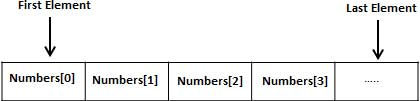C# 基础篇

C# 高级篇

# 数组## 声明数组

``datatype[] arrayName;``

• datatype 用于指定要被存储在数组中的元素的类型
• [ ] 指定数组的大小
• arrayName 指定数组的名称

``double[] balance;``

## 初始化数组

``double[] balance = new double;``

## 赋值数组

``````double[] balance = new double;
balance = 4500.0;``````

``double[] balance = { 2340.0, 4523.69, 3421.0};``

``int [] marks = new int  { 99,  98, 92, 97, 95};``

``int [] marks = new int[]  { 99,  98, 92, 97, 95};``

``````int [] marks = new int[]  { 99,  98, 92, 97, 95};
int[] score = marks;``````

## 访问数组元素

``double salary = balance;``

``````using System;
namespace ArrayApplication
{
class MyArray
{
static void Main(string[] args)
{
int []  n = new int; /* n is an array of 10 integers */
int i,j;

/* initialize elements of array n */
for ( i = 0; i < 10; i++ )
{
n[ i ] = i + 100;
}

/* output each array element's value */
for (j = 0; j < 10; j++ )
{
Console.WriteLine("Element[{0}] = {1}", j, n[j]);
}
}
}
}``````

``````Element = 100
Element = 101
Element = 102
Element = 103
Element = 104
Element = 105
Element = 106
Element = 107
Element = 108
Element = 109``````

## 使用 foreach 循环

``````using System;
namespace ArrayApplication
{
class MyArray
{
static void Main(string[] args)
{
int []  n = new int; /* n is an array of 10 integers */

/* initialize elements of array n */
for ( int i = 0; i < 10; i++ )
{
n[i] = i + 100;
}

/* output each array element's value */
foreach (int j in n )
{
int i = j-100;
Console.WriteLine("Element[{0}] = {1}", i, j);
i++;
}
}
}
}``````

``````Element = 100
Element = 101
Element = 102
Element = 103
Element = 104
Element = 105
Element = 106
Element = 107
Element = 108
Element = 109``````

## 数组详解

Array 类 定义在系统命名空间中，它是基类所有的数组，并使用数组提供了各种属性和方法